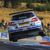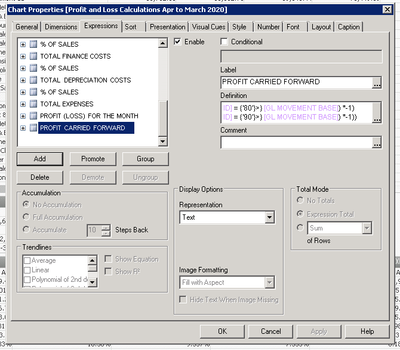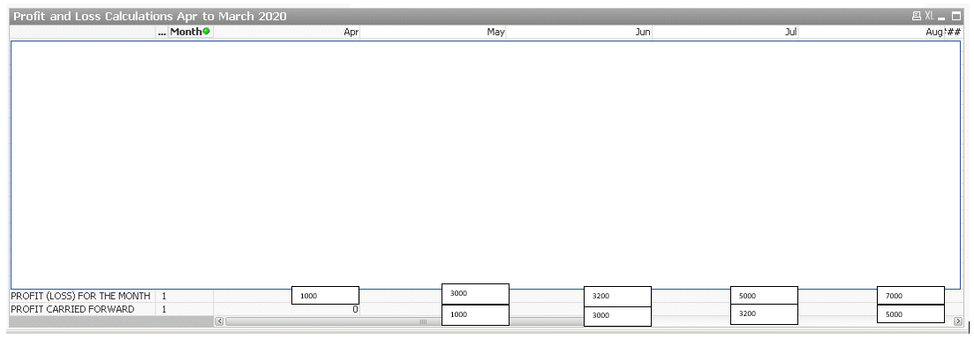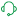# QlikView App Dev

Discussion Board for collaboration related to QlikView App Development.

Announcements
Customer & Partners, DEC. 9, 11 AM ET: Qlik Product & Strategy Roadmap Session: Data Analytics REGISTER NOW
cancel
Showing results for
Did you mean:Creator

## Accumulation Option disabled in Pivot Table

Hi,

Can anyone please explain why Accumulation Option is disabled in a Pivot Table?Can anyone please assist in getting accumulation working? I need to create a running total for profit (loss) within a month in a P&L sheet.

The calculation is as follows to work it out for the current month:

=(((sum({<[SUB CATEGORY ID] = {'10'}>} [GL MOVEMENT BASE]) *-1)
+ (sum({<[SUB CATEGORY ID] = {'20'}>} [GL MOVEMENT BASE]) *-1))
- (sum({<[SUB CATEGORY ID] = {'30'}>} [GL MOVEMENT BASE]) *-1))
+ ((sum({<[SUB CATEGORY ID] = {'40'}>} [GL MOVEMENT BASE]) *-1)
+ (sum({<[SUB CATEGORY ID] = {'50'}>} [GL MOVEMENT BASE]) *-1)
+ (sum({<[SUB CATEGORY ID] = {'60'}>} [GL MOVEMENT BASE]) *-1)
+ (sum({<[SUB CATEGORY ID] = {'70'}>} [GL MOVEMENT BASE]) *-1)
+ (sum({<[SUB CATEGORY ID] = {'80'}>} [GL MOVEMENT BASE]) *-1)
+ (sum({<[SUB CATEGORY ID] = {'90'}>} [GL MOVEMENT BASE]) *-1))

Let's say this total is 2000.

The next month, should be the following calculation:

=(((sum({<[SUB CATEGORY ID] = {'10'}>} [GL MOVEMENT BASE]) *-1)
+ (sum({<[SUB CATEGORY ID] = {'20'}>} [GL MOVEMENT BASE]) *-1))
- (sum({<[SUB CATEGORY ID] = {'30'}>} [GL MOVEMENT BASE]) *-1))
+ ((sum({<[SUB CATEGORY ID] = {'40'}>} [GL MOVEMENT BASE]) *-1)
+ (sum({<[SUB CATEGORY ID] = {'50'}>} [GL MOVEMENT BASE]) *-1)
+ (sum({<[SUB CATEGORY ID] = {'60'}>} [GL MOVEMENT BASE]) *-1)
+ (sum({<[SUB CATEGORY ID] = {'70'}>} [GL MOVEMENT BASE]) *-1)
+ (sum({<[SUB CATEGORY ID] = {'80'}>} [GL MOVEMENT BASE]) *-1)
+ (sum({<[SUB CATEGORY ID] = {'90'}>} [GL MOVEMENT BASE]) *-1))

Let's say this total is 3000

+ the calculation of the previous month (2000), so the total that should show in the table would be 5000.

Make sense?

Thank you in advance for any help.

2 RepliesCreator
Author

Just an update, I've managed to get the totals to move across, using this code here that I found on the forum. I've put this into an expression within my pivot table:

=RangeSum(Alt(Before((((sum({<[SUB CATEGORY ID] = {'10'}>} [GL MOVEMENT BASE]) *-1)
+ (sum({<[SUB CATEGORY ID] = {'20'}>} [GL MOVEMENT BASE]) *-1))
- (sum({<[SUB CATEGORY ID] = {'30'}>} [GL MOVEMENT BASE]) *-1))
+ ((sum({<[SUB CATEGORY ID] = {'40'}>} [GL MOVEMENT BASE]) *-1)
+ (sum({<[SUB CATEGORY ID] = {'50'}>} [GL MOVEMENT BASE]) *-1)
+ (sum({<[SUB CATEGORY ID] = {'60'}>} [GL MOVEMENT BASE]) *-1)
+ (sum({<[SUB CATEGORY ID] = {'70'}>} [GL MOVEMENT BASE]) *-1)
+ (sum({<[SUB CATEGORY ID] = {'80'}>} [GL MOVEMENT BASE]) *-1)
+ (sum({<[SUB CATEGORY ID] = {'90'}>} [GL MOVEMENT BASE]) *-1))),0,))

So, my output now looks like so:However, as you can see, it's showing 0 for April where I would have expected to see 1000.

For the following months, it hasn't added on the previous month, it's just taken the previous month value. I.e. the value of 1000 for April has been put into May where I would have expected to see 4000.

Any ideas?

I've had to change the figures and hide the data due to confidentiality and sensitivity of data.Support (Former)

Check the following Design Blog post to see if that helps you get what you need:

https://community.qlik.com/t5/Qlik-Design-Blog/Accumulations/ba-p/1466581

Regards,
Brett

To help users find verified answers, please do not forget to use the "Accept as Solution" button on any post(s) that helped you resolve your problem or question.
I now work a compressed schedule, Tuesday, Wednesday and Thursday, so those will be the days I will reply to any follow-up posts.Community Browser